top of page
Search

# NumPy Part 2

## Array indexing

1. NumPy mainly offers three ways to index into arrays.

• Slicing Based Indexing

• Integer Based Indexing

• Boolean Based Indexing

### Accessing a single element

• Use [] for accessing the elements inside the array

Example 1*** index starts from 0

## Slicing Based Indexing

• Similar to Python lists, numpy arrays can be sliced. As elements inside the arras are indexed

• Since arrays may be multidimensional, you must specify a slice for each dimension of the array

• A slice of an array is a view into the same data, so modifying it will modify the original array

• When you index into numpy arrays using slicing, the resulting array view will always be a subarray of the original array.

### Accessing multiple element from 2d array

• In 2 dimensional we have rows as one dimension and columns as other dimension (Matrix)

• Rows and Columns index starts from 0• So whenever we are doing slicing we have to provide slicing for both rows as well as for columns*** ending index not included

Example 2

1 ROW AND 1 COLUMNExample 3

"N" ROWS AND "M" COLUMNS### Integer array indexing

• Integer array indexing allows you to construct arbitrary arrays using the data from another array

• Means no change in the original array if we do any change in the sub array

• yield an array of lower rank than the original array

• Since arrays may be multidimensional, you must specify an Integer array indexing for each dimension of the arrayExample 4### Boolean Based indexing

• Boolean array indexing lets you pick out arbitrary elements of an array

• Means no change in the original array if we do any change in the extracted array

• This type of indexing is used to select the elements of an array that satisfy some condition

• It can also be called masking

Example 5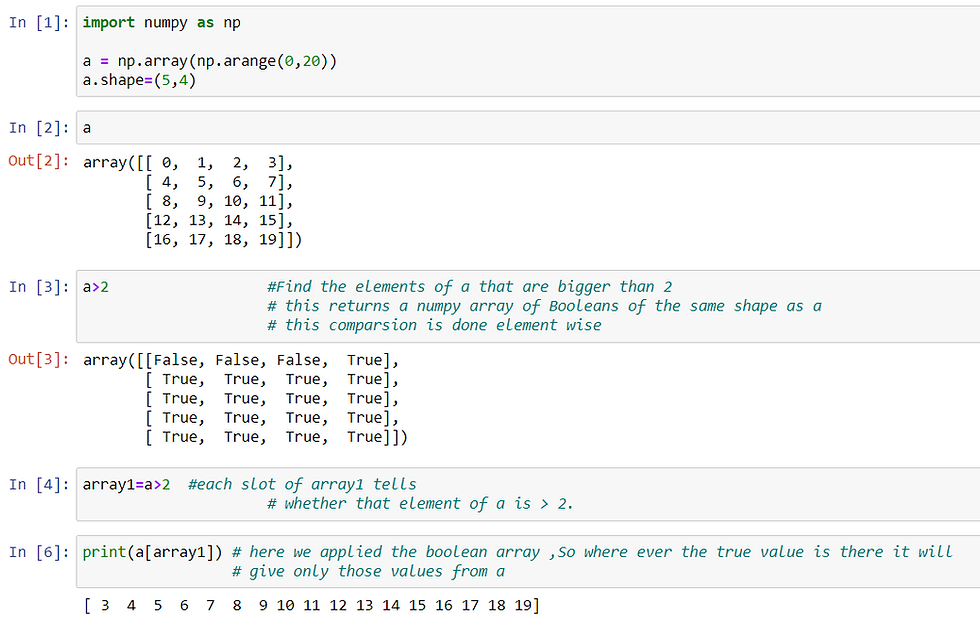Example 6## Mathematical Operations on Arrays

• Basic mathematical functions can be applied on arrays

• This mathematical functions operate elementwise on arrays

• This mathematical operation are available as both as operators and function in numpy module

• We can use + operator to do elementwise addition

• Or we can use add() functions in the NumPy module

Example 7### Subtraction (- or subtract() )

• We can use - operator to do elementwise subtraction

• Or we can use subtract() functions in the NumPy module

Example 8### Multiplication (* or multiply() )

• We can use * operator to do elementwise multiplication

• Or we can use multiply() functions in the NumPy module

Example 9### dot() (to get inner product)

• * is elementwise multiplication, not matrix multiplication

• To do matrix multiplication we have to use dot() function provided by numpy module

• dot() functions computes the inner products

• Whenever we are using this dot() function we have to follow matrix multiplication to rule

• For matrix multiplication, the number of columns in the first matrix must be equal to the number of rows in the second matrix.

• The resulting matrix, has the number of rows of the first and the number of columns of the second matrix as its final shape

• So if you don't follow the above rule then we will get an error

Example 10Example 11• Broadcasting is a powerful mechanism that allows numpy to work with arrays of different shapes when performing arithmetic operations.

• Frequently we have a smaller array and a larger array, and we want to use the smaller array multiple times to perform some operation on the larger array

• whenever we are doing computation on different shapes of array then numpy automatically uses this broadcasting technique

• Broadcasting in a simple way we can understand it as duplicating the rows or column to match the shape of the larger array

• In the below figure we have an array having a shape (3,3) means 3 rows and 3 columns and other array having a shape of (3,) means a single row having 3 elements so when we do multiplication on both the arrays the array with shape (3,) will duplicate the rows 2 times to match the larger array shaped as (3,3)Example 11Example 12Example 13### Division (/ or divide() )

• We can use / operator to do elementwise division

• Or we can use divide() functions in the NumPy module

Example 14### Square Root (sqrt() )

• We can use sqrt() function to get square root of each element in an array

Example 15### sum(array object , axis)

• We can use sum() function to get sum of all elements

• axis (its and optional parameter) parameters has two values 0 (column wise sum) , 1 (rows wise sum)

• If we don't use axis parameter then the sum function will return sum of all the elements in the array

Example 16### prod(array object , axis)

• We can use prod() function to get product of all elements

• axis (its and optional parameter) parameters has two values 0 (column wise product) , 1 (rows wise product)

• If we don't use axis parameter then the prod function will return product of all the elements in the array

Example 17### cumsum(array object , axis)

• We can use cumsum() function to get the cumulative sum of the elements .

• axis (its and optional parameter) parameters has two values 0 (column wise cumulative sum ) , 1 (rows wise cumulative sum )

Example 18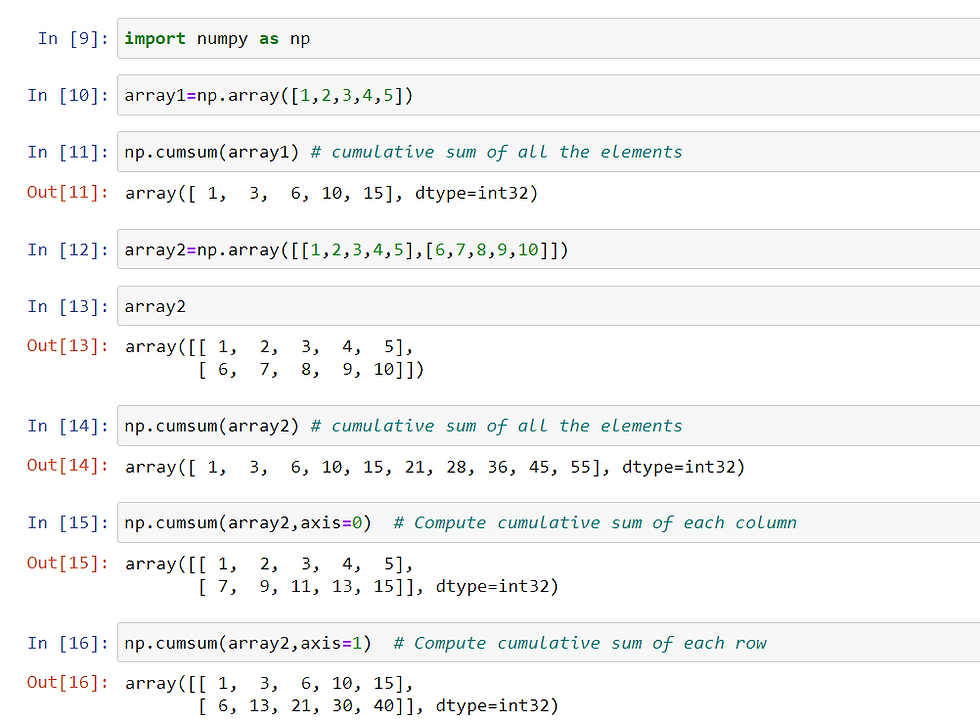### cumprod(array object , axis)

• We can use cumprod() function to get the cumulative product of the elements .

• axis (its and optional parameter) parameters has two values 0 (column wise cumulative product ) , 1 (rows wise cumulative product )

Example 19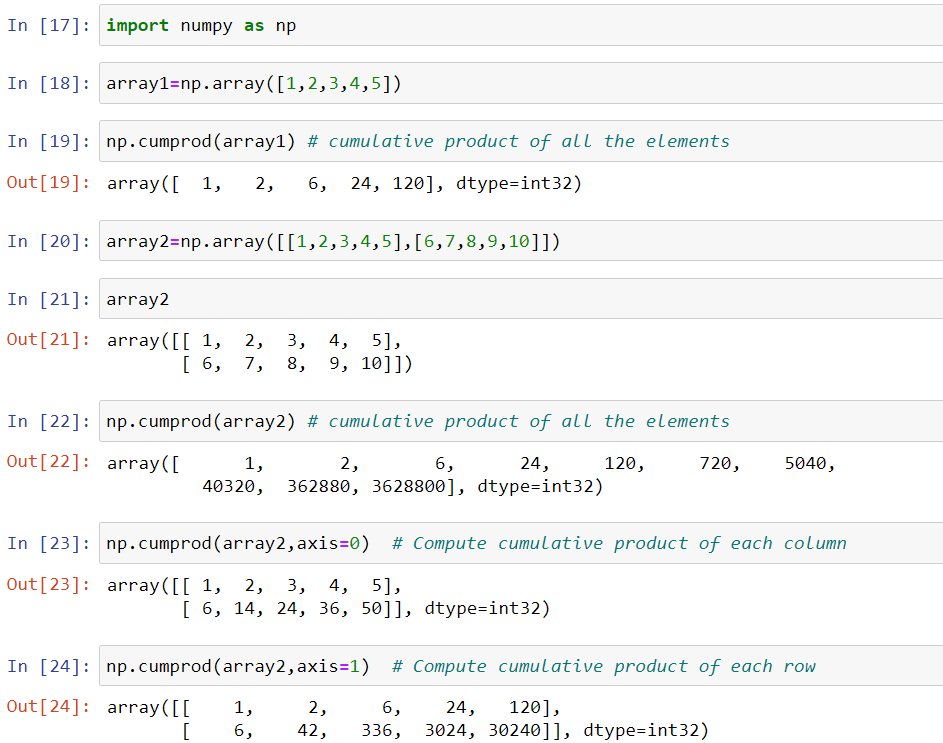### round(array object , decimals)

• Evenly round to the given number of decimals

• decimal parameter is nothing but the Number of decimal places to round to (default: 0)

Example 20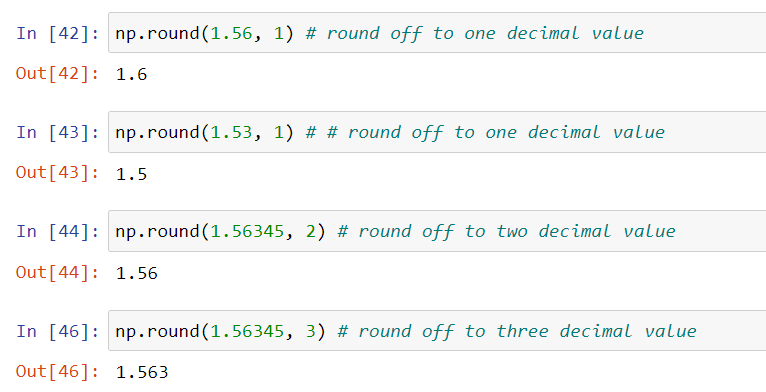### mean()

• Computes the mean of ndarray

• axis parameter can be used to compute mean row wise(1) or column wise(0)

• If axis parameter is not used while computing the mean then mean will be computed for total elements inside the array

Example 21### median()

• Computes the median of ndarray

• axis parameter can be used to compute mean row wise(1) or column wise(0)

• If axis parameter is not used while computing the median then median will be computed for total elements inside the array

Example 22### std() Standard Deviation

• Computes the standard deviation of ndarray

• axis parameter can be used to compute mean row wise(1) or column wise(0)

• If axis parameter is not used while computing the standard deviation then standard deviation will be computed for total elements inside the array

Example 23### min()

• Computes the minimum value of ndarray

• axis parameter can be used to compute mean row wise(1) or column wise(0)

• If axis parameter is not used while computing the minimum value then minimum value will be computed from the total elements inside the array

Example 24### argmin()

• Gets the index of minimum value in the ndarray

Example 24### max()

• Computes the maximum value of ndarray

• axis parameter can be used to compute mean row wise(1) or column wise(0)

• If axis parameter is not used while computing the maximum value then maximum value will be computed from the total elements inside the array

Example 25### argmax()

• Gets the index of maximum value in the ndarray

Example 26## Transpose A Matrix

• We can transpose a matrix(2d) by using T

• It can be also used as a reshape function

• taking the transpose of a rank 1 array does nothing

Example 27## Tile Function

• Tile function is used to create a new array by repeating the array column wise or row wise

• Tile function takes two important arguments

• array

• repetitions along axis (should be given in a tuple format)

• (row wise repetitions ,column wise repetitions)

• (2,3) -- repeat 2 times row wise and three times column wise

Example 28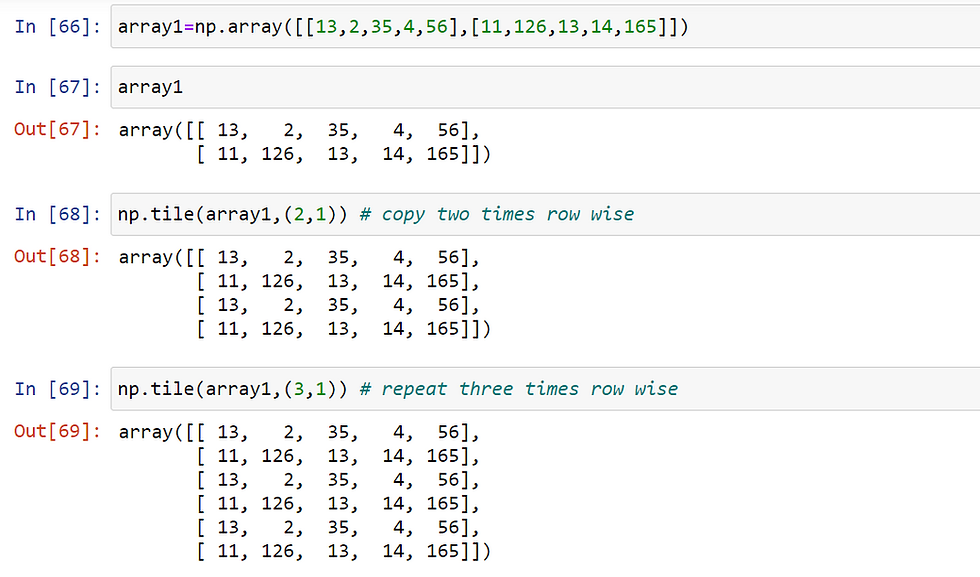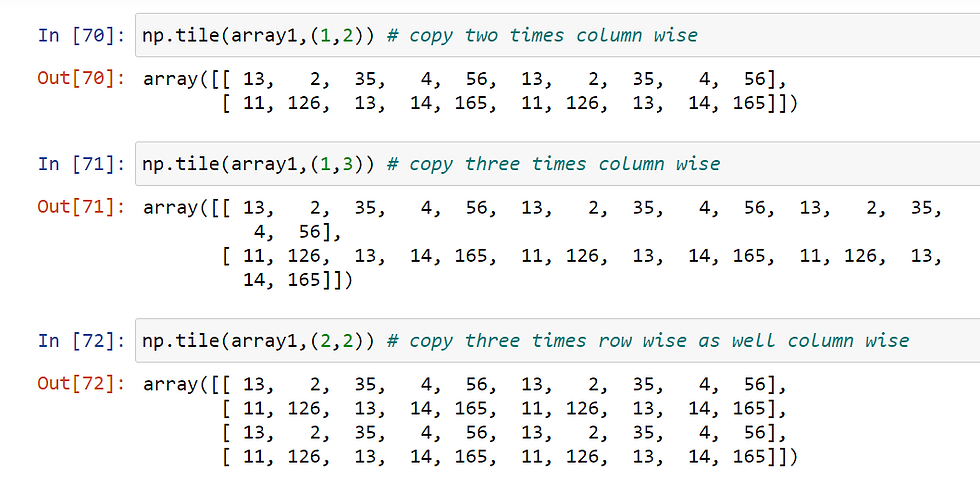## Create An Empty Array With The Same Shape Of Other Array

• We can create an empty array with the same shape of other array by using empty_like()

Example 29